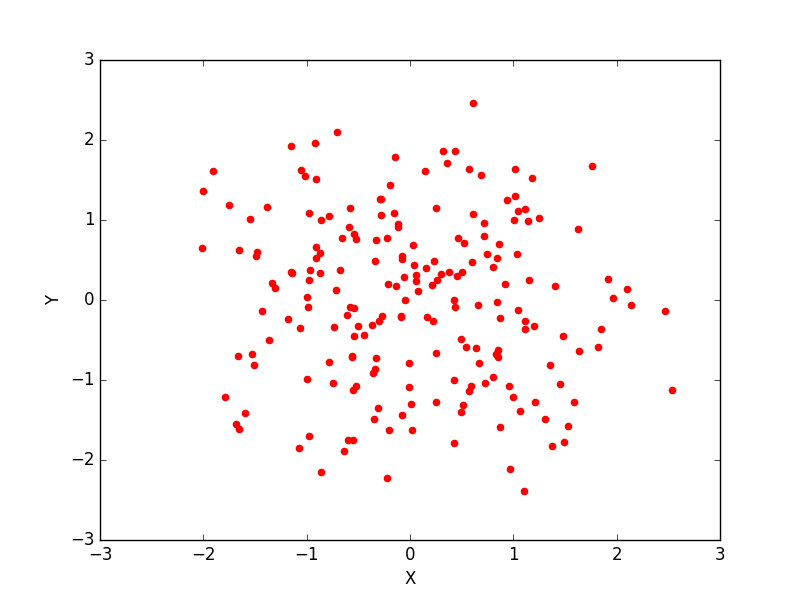﻿ Matplotlib Scatter: Draw a scatter graph taking a random distribution in X and Y - w3resource# Matplotlib Scatter: Draw a scatter graph taking a random distribution in X and Y

## Matplotlib Scatter: Exercise-1 with Solution

Write a Python program to draw a scatter graph taking a random distribution in X and Y and plotted against each other.

Sample Solution:

Python Code:

``````import matplotlib.pyplot as plt
from pylab import randn
X = randn(200)
Y = randn(200)
plt.scatter(X,Y, color='r')
plt.xlabel("X")
plt.ylabel("Y")
plt.show()
```
```

Sample Output:Python Code Editor: